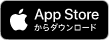## App Storeの説明

The perfect tool for students, teachers and engineers, built on an extremely powerful algebra engine, Symbolic Calculator is capable of helping with any kind of math problems ranging from basic arithmetics to university-level advanced math. It offers all the advantages of an advanced graphing/scientific calculator mixed with the convenience of modern mobile apps.

Features overview:

- Exact arithmetic. 5/15 is 1/3 and not 0.333 (unless you select rounded numerical mode)
- Store unlimited number of variables, create custom functions
- Algebra: operations on polynomials, such as expansion and factorization, collecting terms, division with remainder, etc.
- Calculus: derivatives, integrals, limits, taylor expansion, etc.
- Linear algebra: full support for matrix operations and many useful functions such as: determinant, rank, reduced echelon form, characteristic polynomial, etc.
- Solvers: equations or equation systems, symbolically or numerically, differential equations
- Full support for complex arithmetics
- Plotting
- Session management: export, import sessions or create PDF files
- Many other versatile built-in functions with descriptions and examplesアプリ紹介に戻る

アプリ紹介トップへ